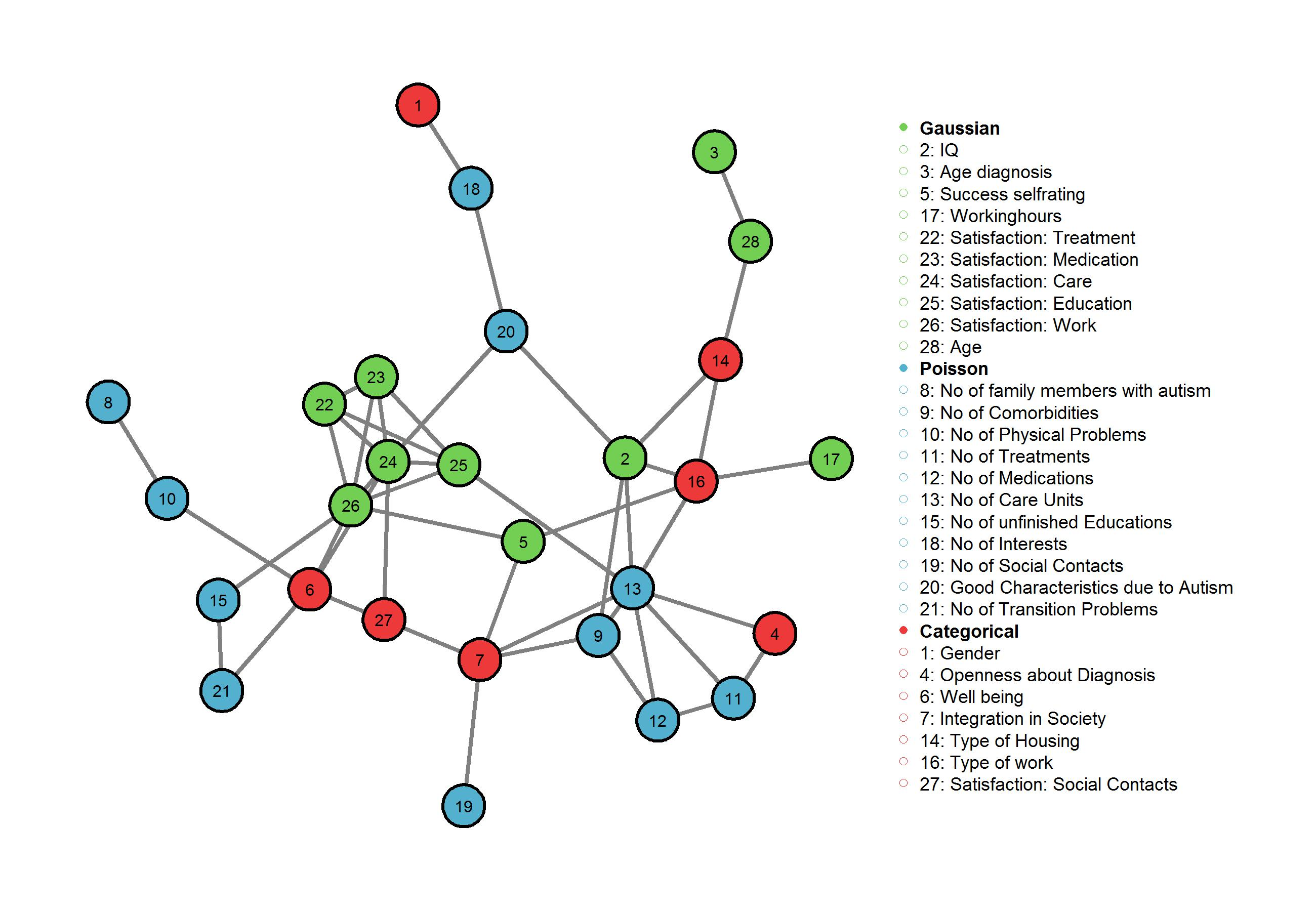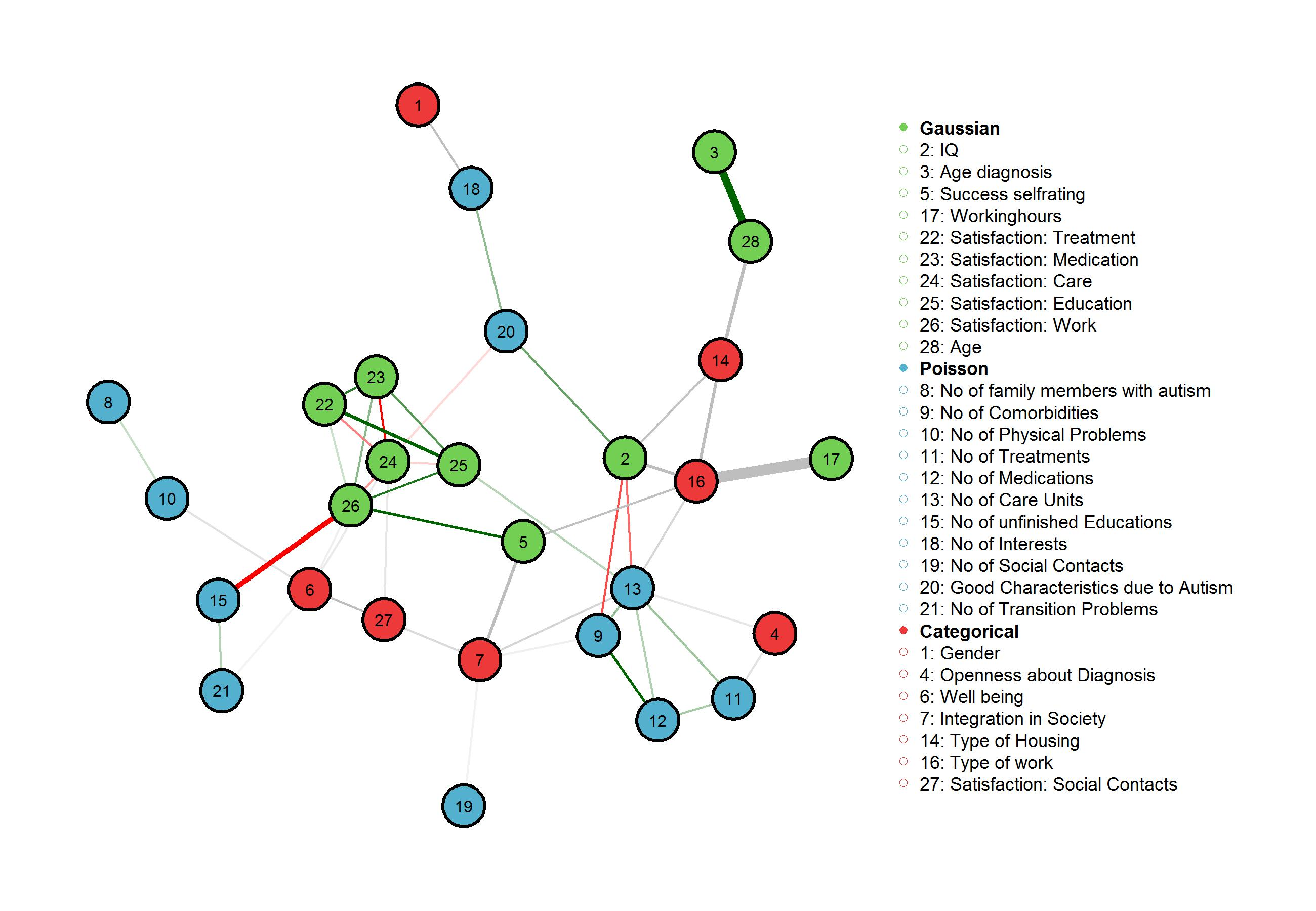Want to share your content on R-bloggers? click here if you have a blog, or here if you don't.

In a previous post we recovered the conditional independence structure in a dataset of mixed variables describing different aspects of the life of individuals diagnosed with Autism Spectrum Disorder, using the mgm package. While depicting the independence structure in multivariate data set gives a first overview of the relations between variables, in most applications we interested in the exact parameter estimates. For instance, for interactions between continuous variables, we would like to know the sign and the size of parameters – i.e., if the nodes in the graph are positively or negatively related, and how strong these associations are. In the case of interactions between categorical variables, we are interested in the signs and sizes of the set of parameters that describes the exact non-linear relationship between variables.

In this post, we take the analysis a step further and show how to use the output of the mgm package to take a closer look at the recovered dependencies. Specifically, we will recover the sign and weight of interaction parameter between continuous variables and zoom into interactions between categorical and continuous variables and between two categorical variables. Both the dataset and the code are available on Github.

We start out with the conditional dependence graph estimated in the previous post, however, now with variables grouped by their type:We obtained this graph by fitting a mixed graphical model using the mgmfit() function as in the previous post:

## Display Edge Weights and Signs

We now also display the weights of the dependencies. In addition, for interactions between continuous (Gaussian, Poisson) variables, we are able determine the sign of the dependency, as it only depends on one parameter. The signs are saved in fit\$signs. To make plotting easier, there is also a matrix fit\$edgecolor, which gives colors to positive (green), negative (red) and undefined (grey) edge signs.

Now, to plot the weighted adjacency matrix with signs (where defined), we give fit\$edgecolor as input to the argument edge.color in qgraph and plot the weighted adjacency matrix `fit\$wadj` instead of the unweighted adjacency matrix `fit\$adj`:

This gives us the following figure:Red edges correspond to negative edge weights and green edge weights correspond to positive edge weights. The width of the edges is proportional to the absolut value of the parameter weight. Grey edges connect categorical variables to continuous variables or to other categorical variables and are computed from more than one parameter and thus we cannot assign a sign to these edges.

While the interaction between continuous variables can be interpreted as a conditional covariance similar to the well-known multivariate Gaussian case, the interpretation of edge-weights involving categorical variables is more intricate as they are comprised of several parameters.

## Interpretation of Interaction: Continuous - Categorical

We first consider the edge weight between the continuous Gaussian variable ‘Working hours’ and the categorical variable ‘Type of Work’, which has the categories (1) No work, (2) Supervised work, (3) Unpaid work and (4) Paid work. We get the estimated parameters behind this edge weight from the matrix of all estimated parameters in the mixed graphical model `fit\$mpar.matrix`:

`fit\$par.labels` indicates which parameters in `fit\$mpar.matrix` belong to the interaction between which two variables. Note that in the case of jointly Gaussian data, `fit\$mpar.matrix` is equivalent to the inverse covariance matrix and each interaction would be represented by 1 value only.

The four values we got from the model parameter matrix represent the interactions of the continuous variable ‘Working hours’ with each of the categories of ‘Type of work’. These can be interpreted in a straight forward way of incraesing/decreasing the probability of a category depending on ‘Working hours’. We see that the probability of category (a) ‘No work’ is greatly decreased by an increase of ‘Working hours’. This makes sense as somebody who does not work has to work 0 hours. Next, working hours seem not to predict the probability of categories (b) ‘Supervised work’ and (c) ‘Unpaid work’. However, increasing working hours does increase the probabilty of category (d) ‘Paid work’, which indicates that individuals who get paid for their work, work longer hours. Note that these interactions are unique in the sense that the influence of all other variables is partialed out!

## Interpretation of Interaction: Categorical - Categorical

Next we consider the edge weight between the categorical variables (14) ‘Type of Housing’ and the variable (15) ‘Type of Work’ from above. ‘Type of Housing’ has to categories, (a) ‘Not independent’ and (b) ‘Independent’. As in the previous example, we take the relevant parameters from the model parameter matrix:

Again, the rows represent the categories of variable (14) ‘Type of Housing’. The columns indicate how the different catgories of variable (16) ‘Type of Work’ predict the probability of these categories. The first column is the dummy category ‘No work’. The parameters can therefore be interpreted as follows:

Having supervised or unpaid work, does not predict a probability of living independently or not that is different for individuals with no work. Having paid work, however, decreases the probability of living not independently and increases the probability of living independently, compared to the reference category ‘no work’.

The interpretations above correspond to the typical interpretation of parameters in a multinomial regression model, which is indeed what is used in the node wise regression approach we use in the mgm packge to estimate mixed graphical models. For details about the exact parameterization of the multinomial regression model check chapter 4 in the glmnet paper. Note that because we use the node wise regression approach, we could also look at how the categories in (16) ‘Type of work’ predict (17) ‘Working hours’ or how the categories of (14) ‘Type of housing’ predict the probabilities of (16) ‘Type of Housing’. These parameters can be obtained by exchanging the row indices with the column indices when subsetting `fit\$mpar.matrix`. For an elaborate explanation of the node wise regresssion approach and the exact structure of the model parameter matrix please check the mgm paper.